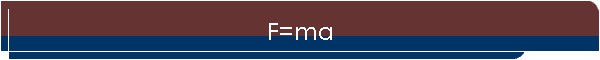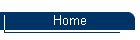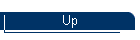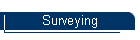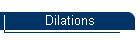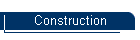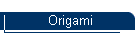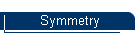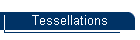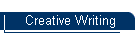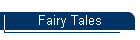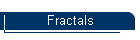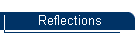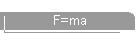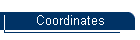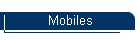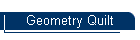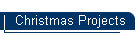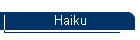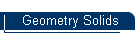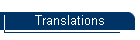DDN

### Pre-calculus Physics Project: posted 2/18/03

#### Force = Mass x Acceleration !

Students calculated the mass of a pickup by measuring the force required to push it, and the resulting acceleration.

##### Mass = Force / Acceleration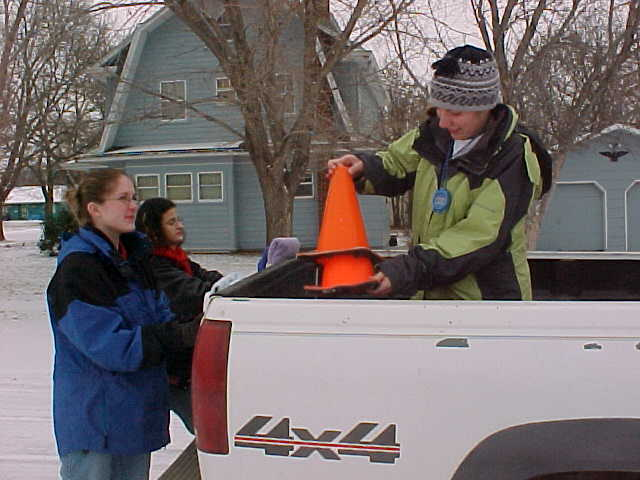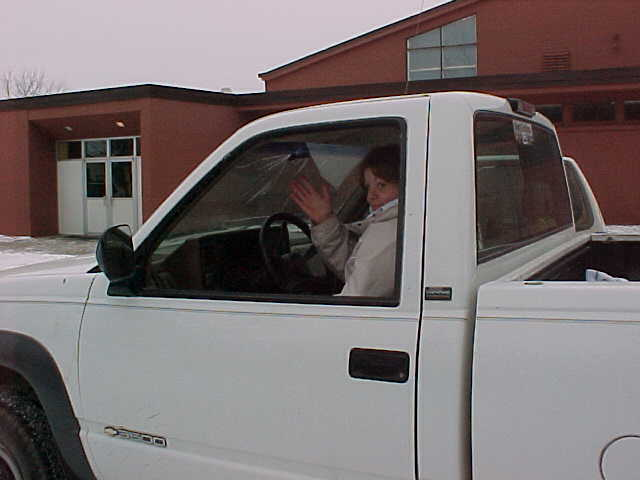Force was held constant by using bathroom scales at 20 lbs. per student pushing.

##### F = 3 pushers x 20 lbs x (1 kg/ 2.2 lbs) x (9.8 m/s/s) = 267 N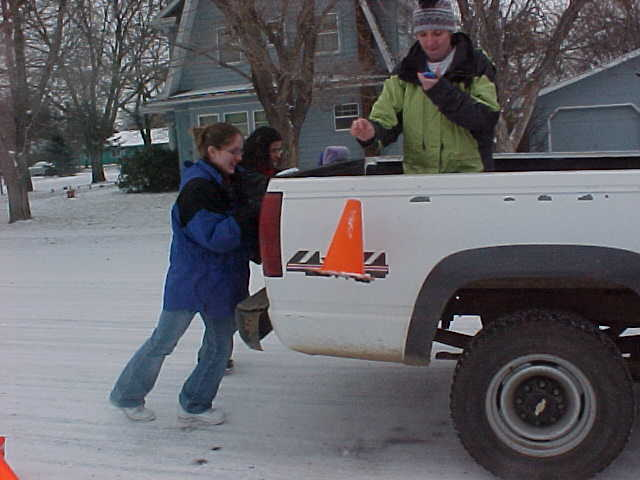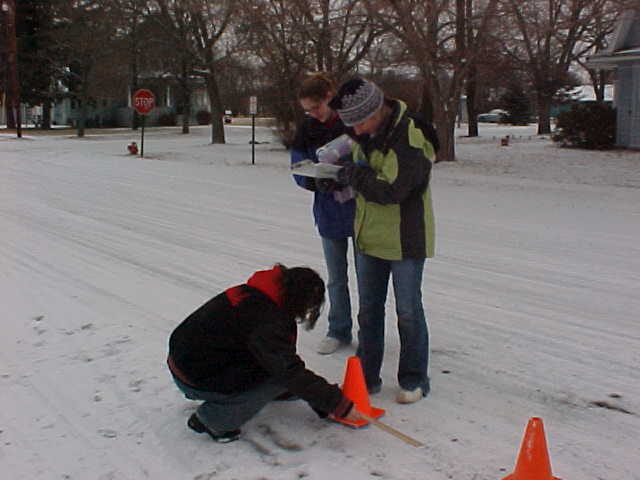Acceleration was measured by marking the pickup's location at 3 second intervals.

(meters)

(meters/sec)

1 0 0
2 1.56 0.52
3 2.22 0.74
4 2.06 0.69
5 2.82 0.94
6 2.89 0.94
7 3.00 1.00
8 2.84 0.95
9 2.23 0.74
10 2.34 0.78
11 2.36 0.79
##### Acceleration

meters/s/s

1 0
2 0.52
3 0.22
4 -0.05
5 0.25
6 0.02
7 0.04
8 -0.05
9 -0.21
10 0.04
11 0.01

Accelerations were averaged to find a mean value for A.  ( A = 0.08 m/s/s )

##### Weight = 3336 kg x (2.2 lbs/kg) =7054 lbs

The student drove to the local grain elevator and had the pickup weighed.

The official result was 6640 lbs.

##### % Error = ((7054 - 6640) / 6640 ) x 100 = +6.2%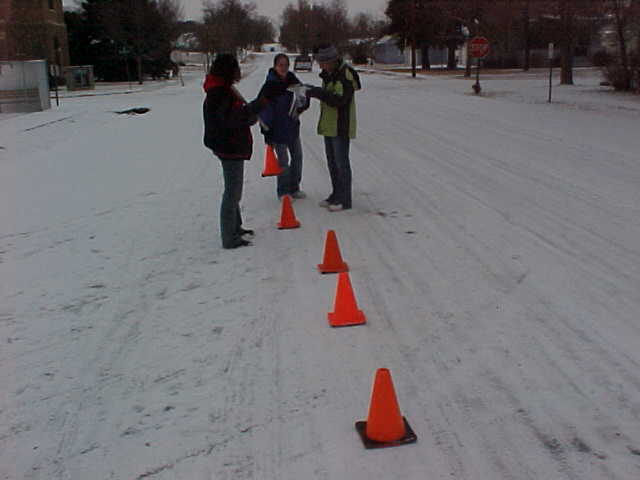Students completed calculations and wrote an essay about the experience.